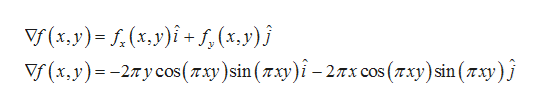# Eavluate the gradient vector at the given point.a) f(x,y) = cos2(πxy) at (1,-1)b) f(x,y,z) = xeyz at (2,1,0)

Question

Eavluate the gradient vector at the given point.

a) f(x,y) = cos2(πxy) at (1,-1)

b) f(x,y,z) = xeyz at (2,1,0)

check_circle

Step 1

a) Given function is

Step 2

Firstly, we find partial derivatives of f with respest to x and y

Step 3

Now, gradient vector of f(x,y) at...help_outlineImage TranscriptioncloseУ(х,у) - 1.(х.у)і +s, (х,y)j V(х.у) -- 2лусos (тху)sin (тxу)i - 2лх сos (тху)sin (тху) j fullscreen

### Want to see the full answer?

See Solution

#### Want to see this answer and more?

Solutions are written by subject experts who are available 24/7. Questions are typically answered within 1 hour.*

See Solution
*Response times may vary by subject and question.
Tagged in

### Derivative